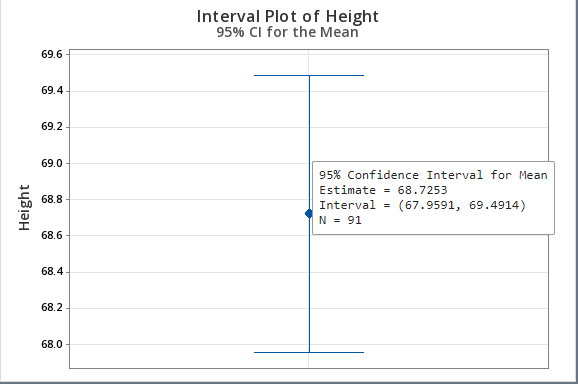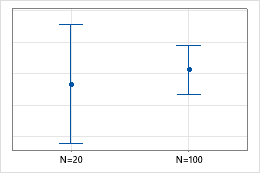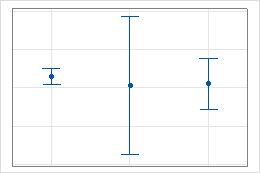# Interpret the key results for Interval Plot

Complete the following steps to interpret an interval plot.

## Step 1: Assess the key characteristics

Examine the center of the distribution. Assess how the sample size may affect the appearance of the interval plot.

### Center

Examine the following elements to learn more about the center of your sample data.
Confidence interval
The confidence interval is a range of values that is likely to include the population mean.
Sample mean
The sample mean is represented by a symbol.

Hold the pointer over the interval to view a tooltip that displays the estimated mean, the confidence interval, and the sample size. For example, this interval plot represents the heights of students. The tooltip indicates that you can be 95% confident that the mean of the heights is between 67.9591 and 69.4914.Investigate confidence intervals that are surprising or unexpected. For example, if a confidence interval for the mean of a sample of customer wait times is substantially different from the confidence intervals of previous samples, you should try to determine the cause of the difference.

### Sample size (n)

The sample size can affect the appearance of the graph.

For example, although these intervals seem quite different, both of them were created using randomly selected samples of data from the same population.Usually, the larger the sample size, the smaller and more precise the confidence interval. If the confidence interval is too wide, try to collect a larger sample. Large differences in sample sizes between groups (or multiple Y variables) can affect the widths of the intervals, and may produce misleading results. If sample sizes for the groups (or multiple Y variables) are approximately the same, you can be more confident that differences in the widths of the intervals is primarily due to differences in variation.

## Step 2: Assess and compare groups

If your interval plot has groups, assess and compare the center and spread of groups.

### Centers

Determine whether any confidence intervals overlap. If the intervals for two means do not overlap, the population means may be statistically significant.

For example, in the first interval plot, the first and second intervals overlap with each other, but they do not overlap with the third interval. Therefore, the mean of the third group could be significantly different than the means of the other two groups. In the second interval plot, the intervals do not overlap. Therefore, the differences in means could be statistically significant.

To determine whether a difference in means is statistically significant, do one of the following:

### Spreads

Look for differences between the spreads of the groups.

The intervals in this plot have similar centers but different spreads.If you see differences in the widths of the intervals, check the sample sizes. Large differences in sample sizes can cause the interval widths to vary.
###### Tip

To determine whether a difference in interval width is caused by a difference in sample variation or a difference in sample size, double-click on an interval. On the Options tab, click Pool error across groups. This recreates the intervals using the pooled standard deviation instead of the individual standard deviations. Any difference in widths you see now are strictly due to different sample sizes.

To determine whether a difference in spread (variance) is statistically significant, do one of the following:
By using this site you agree to the use of cookies for analytics and personalized content.  Read our policy